# Basic Workflow

## Introduction

This vignette shows the basic workflow of using SHAPforxgboost for interpretation of models trained with XGBoost, a hightly efficient gradient boosting implementation .

library("ggplot2")
library("SHAPforxgboost")
library("xgboost")

set.seed(9375)

## Training the model

Let’s train a small model to predict the first column in the iris data set, namely Sepal.Length.

head(iris)
#>   Sepal.Length Sepal.Width Petal.Length Petal.Width Species
#> 1          5.1         3.5          1.4         0.2  setosa
#> 2          4.9         3.0          1.4         0.2  setosa
#> 3          4.7         3.2          1.3         0.2  setosa
#> 4          4.6         3.1          1.5         0.2  setosa
#> 5          5.0         3.6          1.4         0.2  setosa
#> 6          5.4         3.9          1.7         0.4  setosa

X <- data.matrix(iris[, -1])
dtrain <- xgb.DMatrix(X, label = iris[])

fit <- xgb.train(
params = list(
objective = "reg:squarederror",
learning_rate = 0.1
),
data = dtrain,
nrounds = 50
)

## SHAP analysis

Now, we can prepare the SHAP values and analyze the results. All this in just very few lines of code!

# Crunch SHAP values
shap <- shap.prep(fit, X_train = X)

# SHAP importance plot
shap.plot.summary(shap)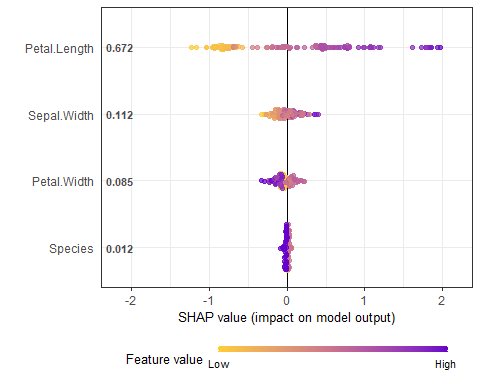# Dependence plots in decreasing order of importance
# (colored by strongest interacting variable)
for (x in shap.importance(shap, names_only = TRUE)) {
p <- shap.plot.dependence(
shap,
x = x,
color_feature = "auto",
smooth = FALSE,
jitter_width = 0.01,
alpha = 0.4
) +
ggtitle(x)
print(p)
}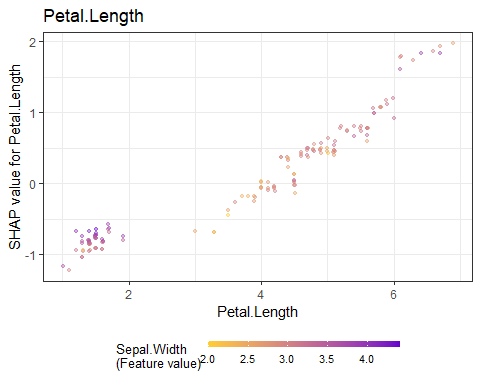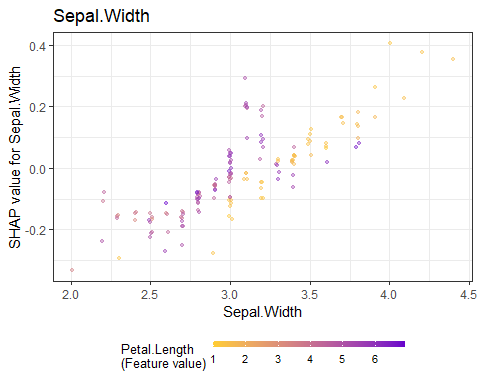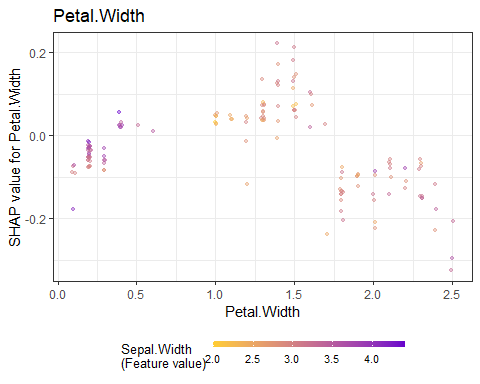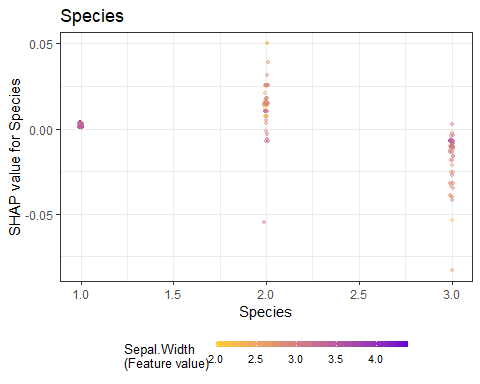Note: print is required only in the context of using ggplot in rmarkdown and for loop.

This is just a teaser: SHAPforxgboost can do much more! Check out the README for much more information.